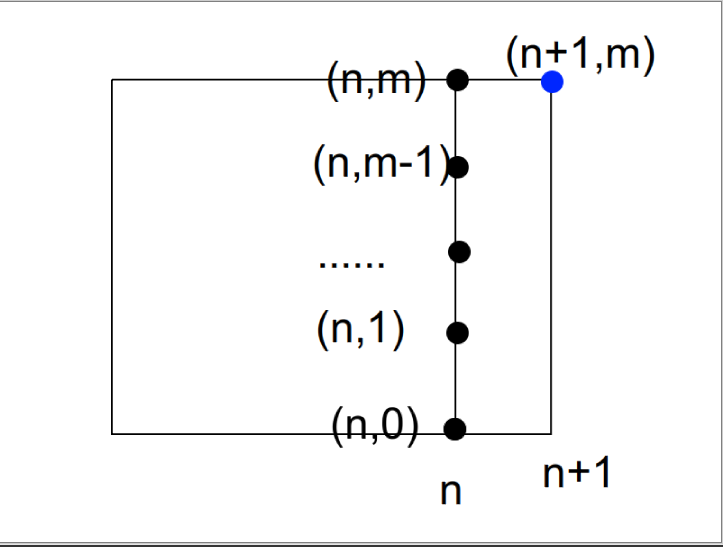# 【瞎总结】组合模型及其组合意义的阐释

## 【总结】组合模型及其组合意义的阐释

> (这种东西)极具启发性，有助于人们深刻理解组合数学。——卢华明

### 可重组合

#### 描述

$m$种球每种球都是足够多的，有$n$个盒子，现在要把盒子塞满(一种球可以用多次)，多少种方案？

#### 公式

$f_0(n,m)={{m+n-1}\choose n}$

#### 拓展套路1

$f_1(n,m)= A_{m+n-1}^{n}={{m+n-1}\choose n}\times n!$

#### 拓展套路2

##### 解法1

$f_2(n,m)=\sum_{i=1}^{n}{n\choose i}{f_0(m,i)}$

##### 解法2

$f_2(n,m)=f_0(n+1,m)$

#### 拓展套路3

$O(2^n)$容斥。

$f_3(n,m)=\sum_{s \subseteq S} (-1)^{|s|}f_2(n+1,m-\sum_{i\in s}(a_i+1))$

#### 拓展套路4(真的拓展)

$\prod_{i=1}^{m}\sum_{j\in S_i}x^j$

$n$项系数

#### 拓展套路5(画蛇添足)

$\prod_{i=1}^{m}\sum_{j=1}^{\inf}x^j=(\frac{x}{1-x})^m$

$n$项系数...

### 不相邻组合

#### 描述

$n$个球，$m$个盒子，(注意看，这里和之前的表述不同了)选出的球不能相邻，有多少种组合方式？

#### 公式

$f_0(n,m)={{n-m+1}\choose{m}}$

#### 组合阐释1

$[0,n-m]$中选出$m$个数，将第$i$小的数加上$i$构成新的序列，新的序列在$[1,n]$的范围内，并且不会重复。

#### 拓展套路1

$\sum_{i=1}^n{n-i+1\choose i}{i+m-1\choose m}$

### 格路模型

${{n+m}\choose n}$

#### 组合阐释2

$dp(i,j)$表示从原点走到$(i,j)$的方案:根据加法原理

$dp(i,j)=dp(i-1,j)+dp(i,j-1)$

${{m+n}\choose n}={{m+n-1}\choose n-1}+{{m+n-1}\choose n}$

$f(n,m)={{m+n}\choose n}$

$f(n,m)=f(n-1,m)+f(n,m-1)$

### 艾提艾斯提模型

${{n+m+1}\choose m}={{n+m}\choose m}+\sum_{i=1}^m {n+m-i\choose m-i}$

#### 组合阐释1(格路)

$\begin{pmatrix} n+m+1\\m\end{pmatrix}$:从原点到$(n+1,m)$的方案数

$\begin{pmatrix} n+m \\ m \end{pmatrix}$:从原点到到$(n,m)$的方案数

$\begin{pmatrix} n+m-i \\ m-i \end{pmatrix}$:从原点到$(n,m-i)$的方案数### 备胎模型

${n \choose l}{l \choose r}={n \choose r}{n-r\choose l-r},l\in[r,n]$

#### 扩展套路1

${n \choose l}{r\choose q}{q\choose m}={n-q\choose l-q}{n-m\choose q-m}{n \choose q}$

$\prod_{i=1}^k{a_{i-1}\choose a_i}={a_0\choose a_k}\prod_{i=1}^{k-1}{a_0-a_i\choose a_i- a_{i+1}}$

### 奇偶模型

$\sum_{i=0} ^n(-1)^i{n \choose i}=[n=0]$

#### 直接证明1

$(x+y)^n= \sum_{i=0}^n {n \choose i}x^iy^{n-i}$

$x=1,y=-1$证毕。

(没有营养的)

### 黑白模型（范德蒙德卷积)

${m+n\choose r} =\sum_{i=0}^r {m\choose i}{n\choose r-i}, r\le m \le n$

#### 拓展套路

$\sum_{i=0}^n{n\choose i}^2={2n\choose n}$

$m=n=r$，并且代入$\begin{pmatrix}a+b\\a\end{pmatrix}=\begin{pmatrix}a+b\\b\end{pmatrix}$即可。

### 下定上动

$\sum_{i=r}^n{i \choose r}={n+1\choose r+1}$

### 下动上定

$\sum_{i=1}^n {n\choose i}=2^n$

#### 直接证明1

$(1+1)^n= \sum_{i=0}^n {n \choose i}1^i1^{n-i}$

### 【小结】$n$球$m$盒：盒子区别？球区别？空盒？

• $n$个球 $m$个盒子 是否空盒 方案数

• 有区别 有区别 有空盒 $m^n$

• 有区别 有区别 无空盒 $m!{\begin{Bmatrix}n \\ \\m\end{Bmatrix}}$ ，不考虑盒子区别时有${\begin{Bmatrix}n \\ \\m\end{Bmatrix}}$种，再乘上$m!$。定义在下

• 有区别 无区别 有空盒 $\sum_i^{\min\{n,m\}} {\begin{Bmatrix}n \\ \\i\end{Bmatrix}}$ ，枚举多少个盒子有，再斯特林数。

• 有区别 无区别 无空盒 ${\begin{Bmatrix}n \\ \\m\end{Bmatrix}}$，套公式

• 无区别 有区别 有空盒 $\text{可重组合}(n,m)=\begin{pmatrix}n+m-1\\ n \end{pmatrix}$

• 无区别 有区别 无空盒 ${\begin{pmatrix}n-1 \\ \\ m-1\end{pmatrix}}$把球排成一列，然后插在$n-1$空里插$m-1$个。

• 无区别 无区别 有空盒 $G(x)=\dfrac 1 {(1-x)(1-x^2)\dots(1-x^{m-1})(1-x^m)}$$x^n$系数

• 无区别 无区别 无空盒 $G(x)=\dfrac 1 {(1-x)(1-x^2)\dots(1-x^{m-1})(1-x^m)}$$x^{n-m}$系数

#### 定义1

$\begin{Bmatrix} n \\ \\ m \end{Bmatrix}$

$\begin{Bmatrix} n \\ \\ m \end{Bmatrix}=\frac 1 {m!}\sum_{i=0}^{m-1} (-1)^i{m \choose i}{(m-i)^n}$

#### 证明1:无区别 无区别 有空盒

• 无区别 无区别 有空盒 $G(x)=\dfrac 1 {(1-x)(1-x^2)\dots(1-x^{m-1})(1-x^m)}$$x^n$系数。

$G(x)=(1+x+x^2+\dots)(1+x^2+x^4+\dots)(1+x^3+x^6+\dots)\dots(1+x^m+x^{2m}+\dots)$

$G(x)={\dfrac {1} {1-x} }{\dfrac {1} {1-x^2} }{\dfrac {1} {1-x^3} }\dots{\dfrac {1} {1-x^m} }$

#### 证明2:无区别 无区别 无空盒

$G(x)={\dfrac {1} {1-x} }{\dfrac {1} {1-x^2} }{\dfrac {1} {1-x^3} }\dots{\dfrac {1} {1-x^m} }$

$x^{n-m}$系数。

### 错排问题

#### 描述

$D_n$表示对于排列$1,2,3,4,5,\dots n$的错排的个数。

#### 递推公式

$1,2,3,\dots n-1,n$

$n$和1到n-1任何一个数k互换，然后剩下的$n-1$个数进行错排。注意到错排个数只和排列长度有关，则有$(n-1)D_{n-1}$方案。但是我们考虑到k永远不会回到k原来的那个位置(被n占了)，所以还有$n-1-1=n-2$个元素进行错排。得到递推

$D_n=(n-1)(D_{n-1}+D_{n-2})$

$D_0=1,D_1=0$

$D_n=B_{n-1}+B_{n-2}+D_{n-2}$

#### 求通项(指数型生成函数)

$\begin{array} & D_n-nD_{n-1} \\ &=-[D_{n-1}-(n-1)D_{n-2}] \\ &=(-1)^2[D_{n-2}-(n-2)D_{n-3}] \\ &= \dots \\ &=(-1)^n(D_2-D_1) \\ &=(-1)^n \end{array}$

$G(x)=D_0+D_1\dfrac 1 {1!}x+\dfrac 1 {2!}D_2x^2+\dfrac 1 {3!}D_3x^3+\dots$

$e^{-x}=\dfrac 1 {0!} -\dfrac 1 {1!} x+\dfrac 1 {2!}x^2-\dfrac 1 {3!}x^3\dots$

$G(x)-xG(x)=e^{-x}$

$\begin{array} &G(x)=\dfrac {e^{-x}} {1-x} \\ =e^{-x}(\dfrac 1 {1-x}) \\ =(\dfrac 1 {0!} -\dfrac 1 {1!} x+\dfrac 1 {2!}x^2-\dfrac 1 {3!}x^3\dots)(1+x+x^2+x^3+\dots) \\ =D_0+D_1\dfrac 1 {1!}x+\dfrac 1 {2!}D_2x^2+\dfrac 1 {3!}D_3x^3+\dots \end{array}$

$[x^n]=\sum_{i=0}^n(-1)^i\dfrac {n!} {i!}=D_n$

$D_n=\sum_{i=0}^n (-1)^i\dfrac {n!} {i!}$

#### 求通项(组合阐释)

$D_n=\sum_{i=0}^n (-1)^i\dfrac {n!} {i!}$

### 分圆排列(第一类斯特林)

#### 描述

$\begin{bmatrix}n \\ m\end{bmatrix}$

#### 递推关系

$\begin{bmatrix}n \\ m\end{bmatrix}=\begin{bmatrix}n-1 \\ m-1\end{bmatrix}+(n-1)\begin{bmatrix}n-1 \\ m\end{bmatrix} \\ {\begin{bmatrix}n \\ n\end{bmatrix}=1},{\begin{bmatrix}n \\ 0\end{bmatrix}=0},n>0$

#### 扩展套路1

$\sum_{i=0}^n {\begin{bmatrix} n \\ i\end{bmatrix}}=n!$

$\begin{matrix} a_1&a_2&a_3&a_4&\dots &a_n\end{matrix}$

$P=\begin{bmatrix}1,2,4,3,6,5\end{bmatrix}$

$\S =\begin{Bmatrix} &&[3,4]&[5,6] \end{Bmatrix}$

### 子集列(第二类斯特林数)

#### 描述

$n$个有区别的球放入$m$个无区别的盒子，盒子非空。上面那个小结里就介绍了第一类斯特林数。

$\begin{Bmatrix}n\\ \\ m\end{Bmatrix}$

#### 递推关系

$\begin{Bmatrix}n\\ \\ m\end{Bmatrix}=\begin{Bmatrix}n-1\\ \\ m-1\end{Bmatrix}+m\begin{Bmatrix}n-1\\ \\ m\end{Bmatrix}$

#### 容斥关系

$\begin{Bmatrix}n\\ \\ m\end{Bmatrix}=\dfrac 1 {m!} \sum_{i=0}^{m-1}(-1)^i{m\choose i}(m-i)^n$

### 反演关系

$i^k=\sum_{j=0}^{\min\{i,k\}} {k\brace j}\begin{pmatrix} i \\j\end{pmatrix}j!$

#### 特殊值和性质

• $\begin{Bmatrix}n\\ \\ 2\end{Bmatrix}=2^n-1,n>0$

• $\begin{Bmatrix}n\\ \\ 3\end{Bmatrix}=\dfrac 1 {3!}(3^n-3\times 2^n+3)=\dfrac {3^{n-1}+1-2^n} {2}$

• $\begin{Bmatrix}n\\ \\ n-1\end{Bmatrix}=\begin{pmatrix}n\\ \\ 2\end{pmatrix}$

• $\begin{Bmatrix}n\\ \\ n-2\end{Bmatrix}=\begin{pmatrix}n\\ \\ 3\end{pmatrix}+3\begin{pmatrix}n\\ \\ 4\end{pmatrix}$

(鸡贼口中的)超级卡特兰数



###### 弦鸽不缀
posted @ 2019-06-10 22:26  谁是鸽王  阅读(591)  评论(6编辑  收藏